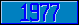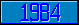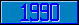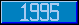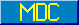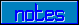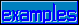## MWAPI - ROWCOL

### 6.4.22 ROWCOL elattribute

 Definition ROWCOL specifies how choices are arranged within rows and columns in a radio button set gadget. If a gadget contains N choices, they are displayed in a matrix of R rows by C columns. If rowcol is equal to "ROW", R is equal to rowcolsize and C is equal to the M[UMPS] numeric interpretation of N\R+(N#R>0) If rowcol is equal to "COL", C is equal to rowcolsize and R is equal to the M[UMPS] numeric interpretation of N\C+(N#C>0) If filldir is equal to "H", then rows are filled first. That is, all cells in row n are filled before any cells in row n+1 are filled. If filldir is equal to "V", then columns are filled first. That is, all cells in column n are filled before any cells in column n+1 are filled. It is possible to have empty cells, rows and columns. If an application attempts to assign a rowcolsize value that is less than 1 (one), an error occurs with \$ECODE containing ",M?2," and \$EREF containing a value that indicates the ssvn reference for which the error occurred. All columns have the same width. If the SIZE elattribute is specified for the gadget, the column width is approximately equal to hsize/C. If the SIZE elattribute is not specified for the gadget, the column width is approximately equal to the widest choice specified for the gadget. Domain rowcolspec Access Create, Reference Default If ROWCOL is not specified it defaults to "COL,1,V". If intlit is not specified, it defaults to 1 (one). If filldir is not specified, it defaults to "V".